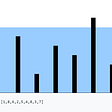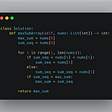# Search in a Sorted Array of Unknown Size — Day 105(Python)

Today’s question is a medium-tagged question. Let’s go!

702. Search in a Sorted Array of Unknown Size

This is an interactive problem.

You have a sorted array of unique elements and an unknown size. You do not have an access to the array but you can use the `ArrayReader` interface to access it. You can call `ArrayReader.get(i)` that:

• returns the value at the `ith` index (0-indexed) of the secret array (i.e., `secret[i]`), or
• returns `2^31 - 1` if the `i` is out of the boundary of the array.

You are also given an integer `target`.

Return the index `k` of the hidden array where `secret[k] == target` or return `-1` otherwise.

You must write an algorithm with `O(log n)` runtime complexity.

Example 1:

`Input: secret = [-1,0,3,5,9,12], target = 9Output: 4Explanation: 9 exists in secret and its index is 4.`

Example 2:

`Input: secret = [-1,0,3,5,9,12], target = 2Output: -1Explanation: 2 does not exist in secret so return -1.`

Constraints:

• `1 <= secret.length <= 10^4`
• `-104 <= secret[i], target <= 10^4`
• `secret` is sorted in a strictly increasing order.

We know that we need to search for an element in a sorted array. This means that we will be using binary search. (Hint: Searching in sorted array = Binary Search).

One additional condition is, we do not know the length of the array that needs to be searched. But we do know the max length of the array possible. Hence we will assume the length of the array is max length.

Alright, let’s look into the code.

`# """# This is ArrayReader's API interface.# You should not implement it, or speculate about its implementation# """#class ArrayReader:#    def get(self, index: int) -> int:class Solution:    def search(self, reader: 'ArrayReader', target: int) -> int:        low = 0        high = pow(10,4)        while(low <= high):            mid = (low + high) //2            out = reader.get(mid)            if(out == target):                return mid            elif(target < out):                high = mid - 1            else:                low = mid + 1        return -1`

Complexity Analysis

Time Complexity

Every time we look for the target on our list, we are dividing our list by half. Hence time complexity is O(logN), where N is the max length.

Space Complexity

We are making use of constant space while we perform binary search and hence space complexity is O(1).

We can solve binary search through recursion too. When we are planning to use recursion, we need to identify the base condition. In this case, our base conditions are when low is greater than high then we would return -1 i.e. we did not find the required number, or we found the target.

Until we reach the Base condition, we keep dividing our array in half. Let us look into the code.

`# """# This is ArrayReader's API interface.# You should not implement it, or speculate about its implementation# """#class ArrayReader:#    def get(self, index: int) -> int:class Solution:    def search(self, reader: 'ArrayReader', target: int) -> int:        def binarysearch(low, high, reader, target):            if(low > high):                return -1            mid = (low + high) //2            out = reader.get(mid)            if(out == target):                return mid            elif(target < out):                high = mid - 1                return binarysearch(low, high, reader, target)            else:                low = mid + 1                return binarysearch(low, high, reader, target)        low = 0        high = pow(10,4)        output = binarysearch(low, high, reader, target)        return output`

Complexity Analysis

Time Complexity

Every time we look for the target on our list, we are dividing our list by half. Hence time complexity is O(logN), where N is the max length.

Space Complexity

Since recursion internally uses stack, space complexity is O(logN).

--

--

--

## More from Annamariya Tharayil

Software Engineer. Find me @ www.linkedin.com/in/annamariya-jt

Love podcasts or audiobooks? Learn on the go with our new app.

## Recommended from Medium## What is Embedded Analytics? How Tableau embeds to Salesforce in hig?## Atlan raised \$16 million in a Series A and released Job Profiles for Team Expansion.## Automated Maintenance for Apache Airflow## How to Create a GDP Per Capita Country Comparison in AR in 15 Minutes or Less [Tutorial]## Automating AWS Backup & Restore with EC2, Lambda, and SNS## Nano Digest — Unreal Nano, Dev Update, RoboCash, Moons and more!## Annamariya Tharayil

Software Engineer. Find me @ www.linkedin.com/in/annamariya-jt

## Remove Nth Node From End of List — Day 107(Python)## Weekly Contest 273 Q4, 2122. Recover the Original Array (Hard)## Leetcode: 11 Container With Most Water (medium)## LeetCode Patterns Adventure 7 — Maximum Subarray